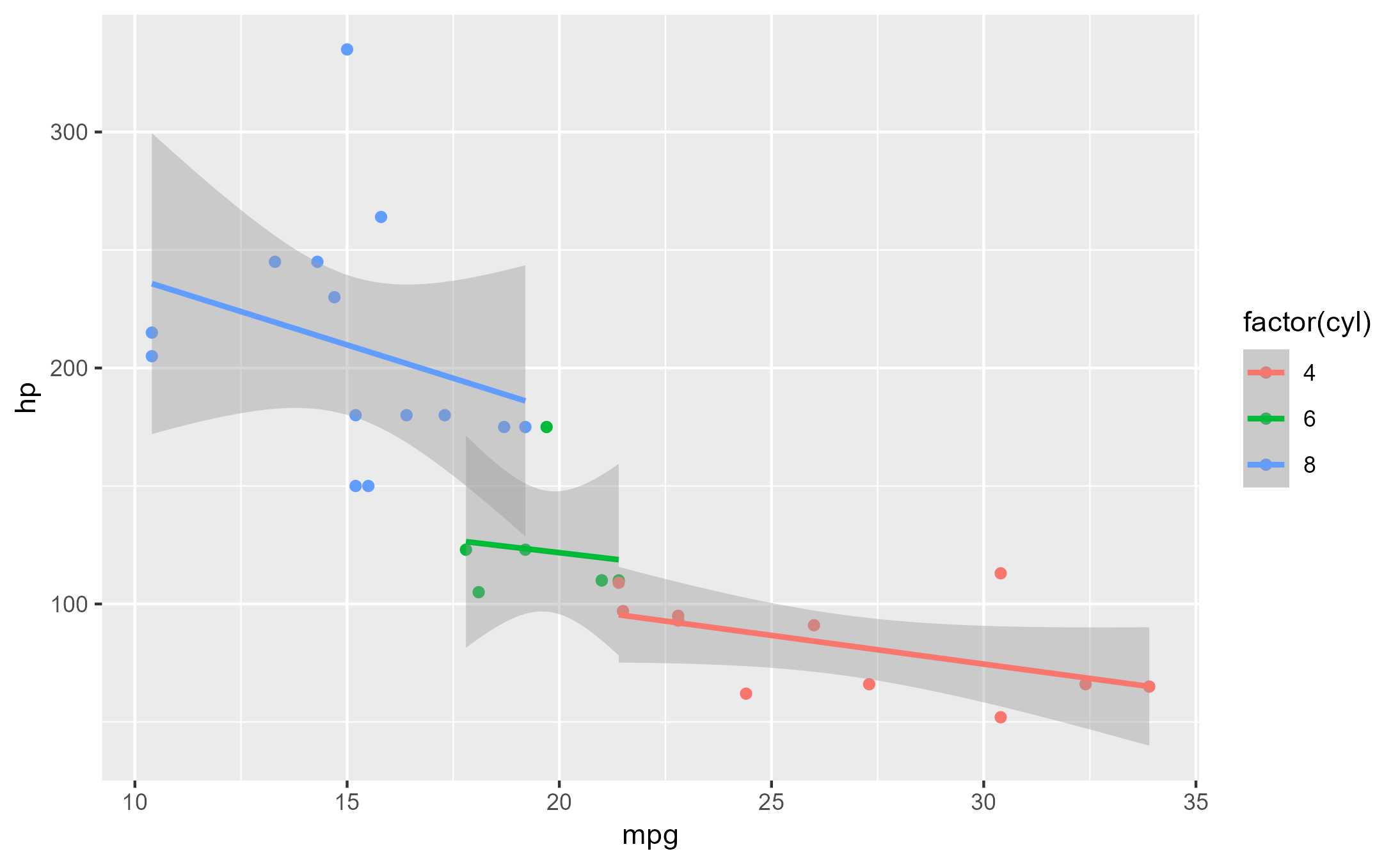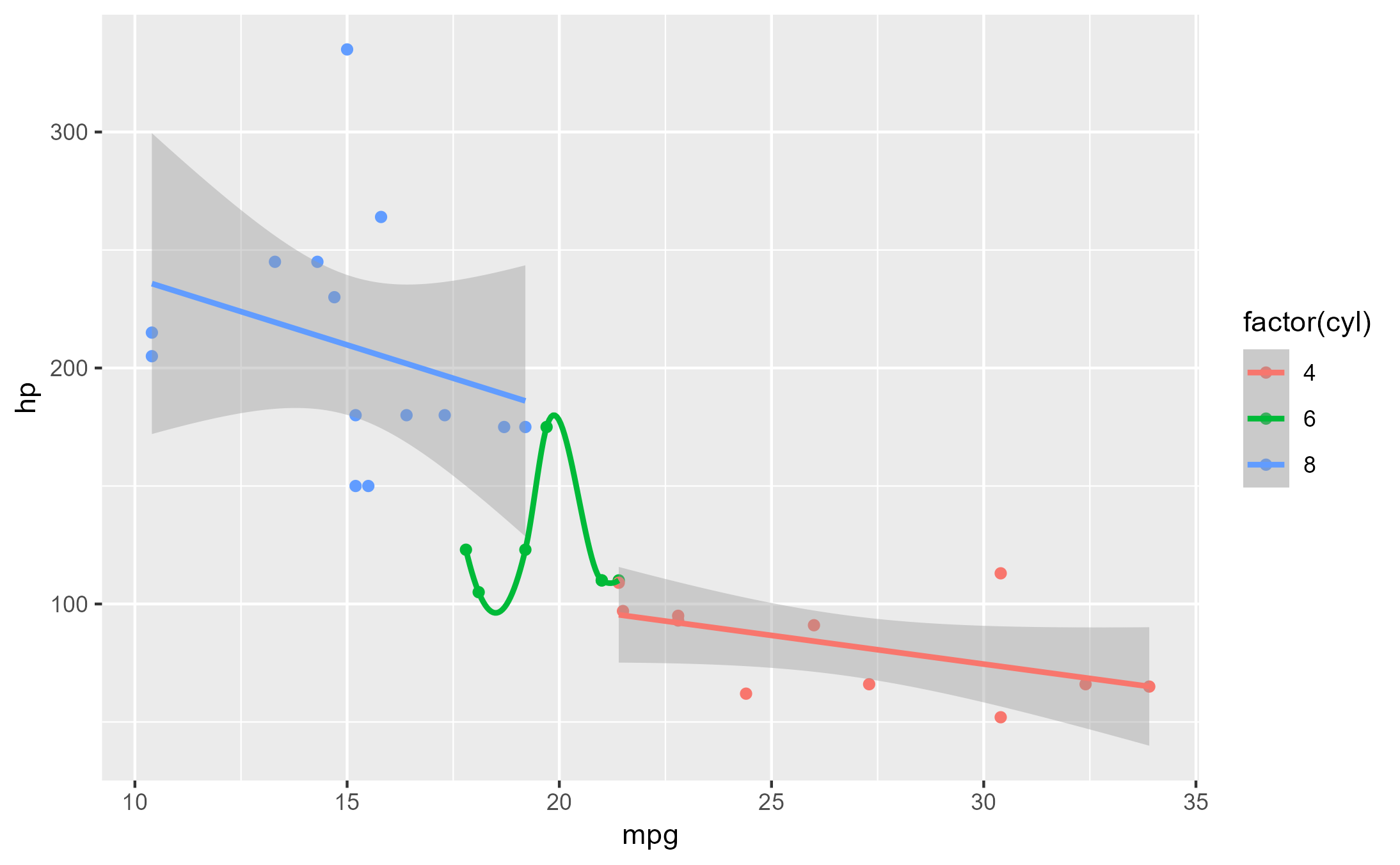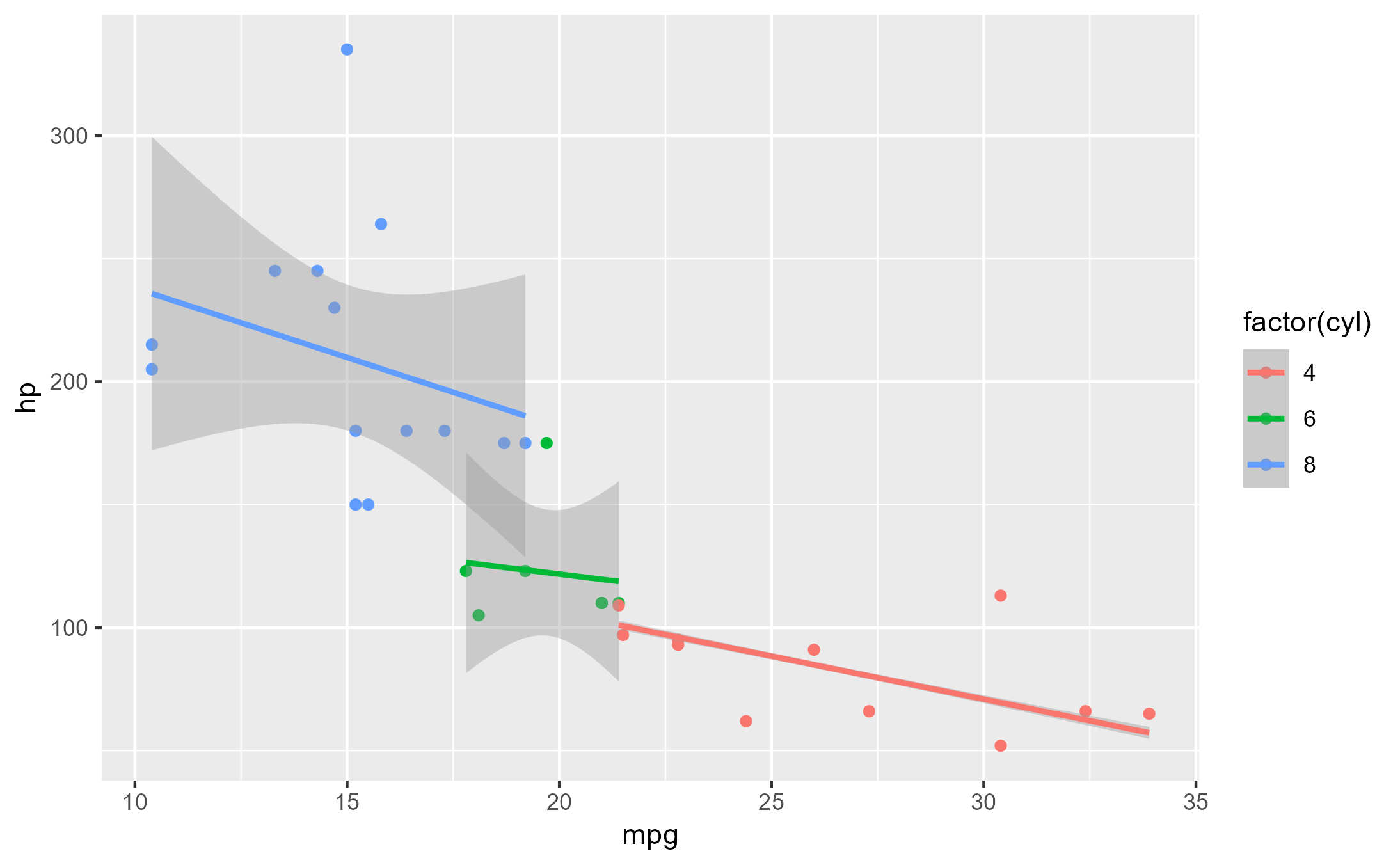Highjack a method's execution and modify its argument values

## Usage

ggtrace_highjack_args(x, method, cond = 1L, values, draw = TRUE)

## Arguments

x

A ggplot object

method

A function or a ggproto method. The ggproto method may be specified using any of the following forms:

• ggproto$method • namespace::ggproto$method

• namespace:::ggproto$method cond When the return value should be replaced. Defaults to 1L. values A named list of variable-value pairings. When values are expressions, they are evaluated in the formals. draw Whether to draw the modified graphical output from evaluating x. Defaults to TRUE. ## Value A gtable object with class <ggtrace_highjacked> ## Tracing context When quoted expressions are passed to the cond or value argument of workflow functions they are evaluated in a special environment which we call the "tracing context". The tracing context is "data-masked" (see rlang::eval_tidy()), and exposes an internal variable called ._counter_ which increments every time a function/method has been called by the ggplot object supplied to the x argument of workflow functions. For example, cond = quote(._counter_ == 1L) is evaluated as TRUE when the method is called for the first time. The cond argument also supports numeric shorthands like cond = 1L which evaluates to quote(._counter_ == 1L), and this is the default value of cond for all workflow functions that only return one value (e.g., ggtrace_capture_fn()). It is recommended to consult the output of ggtrace_inspect_n() and ggtrace_inspect_which() to construct expressions that condition on ._counter_. For highjack functions like ggtrace_highjack_return(), the value about to be returned by the function/method can be accessed with returnValue() in the value argument. By default, value is set to quote(returnValue()) which simply evaluates to the return value, but directly computing on returnValue() to derive a different return value for the function/method is also possible. ## Examples  set.seed(1116) library(ggplot2) library(dplyr) p <- ggplot(mtcars, aes(mpg, hp, color = factor(cyl))) + geom_point() + geom_smooth(method = "lm") p #> geom_smooth() using formula = 'y ~ x'# Fit predictions from loess regression just for second group ggtrace_highjack_args( x = p, method = StatSmooth$compute_group,
cond = quote(data$group == 2), values = list(method = "loess") ) #> geom_smooth() using formula = 'y ~ x'# If value is an expression, it's evaluated in the Tracing Context ggtrace_highjack_args( x = p, method = StatSmooth$compute_group,
values = rlang::exprs(

# Every time the method is called, call it with a bigger CI
level = ._counter_ * 0.3,

# Fit models to just a random sample of the data
data = data %>%
slice_sample(prop = .8)

)
)
#> geom_smooth() using formula = 'y ~ x'